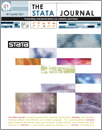Home  >>  Archives  >>  Volume 13 Number 4  >>  st0314

#### The Stata JournalVolume 13 Number 4: pp. 672-698

Subscribe to the Stata Journal## Attributable and unattributable risks and fractions and other scenario comparisons

 Roger B. Newson National Heart and Lung Institute Imperial College London London, UK r.newson@imperial.ac.uk
Abstract.  Scenarios are alternative versions of the same dataset with the same variables but different observations or values. Applied scientists frequently want to predict how much good an intervention will do by comparing outcomes from the same model between different scenarios. Alternatively, they may want to compare outcomes between different models applied to the same scenario, for instance, when standardizing statistics from different subpopulations to a common gender and age distribution. Standard Stata tools for scenario means and comparisons are margins and pwcompare. A suite of packages is presented for estimating scenario means and comparisons by using margins, together with normalizing and variance-stabilizing transformations implemented by using nlcom. margprev estimates marginal prevalences; marglmean estimates marginal arithmetic means; regpar estimates the difference between two marginal prevalences (the population attributable risk); punaf estimates the ratio between two marginal arithmetic means (the population unattributable fraction); and punafcc estimates a marginal mean between-scenario risk or hazard ratio for case–control or survival data (also known as a population unattributable fraction). The population unattributable fraction and its confidence limits are subtracted from 1 to estimate the population attributable fraction. Formulas and examples are presented, including an example from the Global Allergy and Asthma European Network.

View all articles by this author: Roger B. Newson

View all articles with these keywords: margprev, marglmean, regpar, punaf, punafcc, margins, nlcom, population, unattributable, attributable, risk, fraction, PAR, PAF, PUF, scenario, comparison, standardization